Processing ......FreeComputerBooks.com Links to Free Computer, Mathematics, Technical Books all over the World

Model Theory, Algebra, and Geometry
🌠 Top Free Java Books - 100% Free or Open Source!
• Title Model Theory, Algebra, and Geometry
• Authors Deirdre Haskell, Anand Pillay, and Charles Steinhorn
• Publisher: Cambridge University Press (June 10, 2010)
• Paperback: 236 pages
• eBook: PDF Files
• Language: English
• ISBN-10: 0521143497
• ISBN-13: 978-0521143493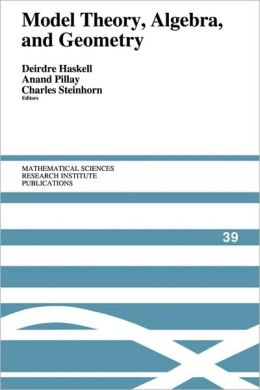Book Description

Model theory is a branch of mathematical logic that has found applications in several areas of algebra and geometry. It provides a unifying framework for the understanding of old results and more recently has led to significant new results, such as a proof of the Mordell-Lang conjecture for function fields in positive characteristic. Perhaps surprisingly, it is sometimes the most abstract aspects of model theory that are relevant to those applications.

This book gives the necessary background for understanding both the model theory and the mathematics behind the applications. Aimed at graduate students and researchers, it contains introductory surveys by leading experts covering the whole spectrum of contemporary model theory (stability, simplicity, o-minimality and variations), and introducing and discussing the diverse areas of geometry (algebraic, diophantine, real analytic, p-adic, and rigid) to which the model theory is applied.

The book begins with an introduction to model theory. It then broadens into three components: pure model theory, geometry, and the model theory of fields.

• N/A
Reviews, Ratings, and Recommendations: Related Book Categories: Read and Download Links:Similar Books:
•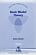Basic Model Theory (Kees Doets)

As the title indicates, this book introduces the reader to what is basic in model theory. A special feature is its use of the Ehrenfeucht game by which the reader is familiarised with the world of models.

•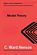Model Theory (C. Ward Henson)

It is an up-to-date textbook of model theory taking the reader from first definitions to Morley's theorem and the elementary parts of stability theory, introduces the model theory of first-order logic, avoiding syntactical issues not too relevant to model theory.

•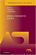Model-Theoretic Logics (Jon Barwise, et al)

This book brings together several directions of work in model theory between the late 1950s and early 1980s. It provides an introduction to the subject as a whole, as well as to the basic theory and examples. Many chapters can be read independently.

•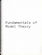Fundamentals of Model Theory (William Weiss, et al)

This book is a concluding discussion focuses on the relationship between proofs and formal derivations, and the role proofs may play as part of a general theory of evidence. It is a primer which will give someone a self contained overview of the subject.

•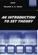An Introduction to Set Theory (William A. R. Weiss)

This book covers the basics: relations, functions, orderings, finite, countable, and uncountable sets, and cardinal and ordinal numbers, gives students sufficient grounding in a rigorous approach to the revolutionary results of set theory.

•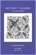Abstract Algebra: Theory and Applications (Thomas W Judson)

This book is designed to teach the principles and theory of abstract algebra to college juniors and seniors in a rigorous manner. Its strengths include a wide range of exercises, both computational and theoretical, plus many nontrivial applications.

•Topology: A Categorical Approach (Tai-Danae Bradley, et al)

A graduate-level textbook that presents basic topology from the perspective of category theory. Many graduate students are familiar with the ideas of point-set topology and they are ready to learn something new about them.

Book Categories
 :All CategoriesTop Free BooksRecent BooksMiscellaneous BooksComputer EngineeringComputer LanguagesComputer ScienceData Science/DatabasesJava and Java EE (J2EE)Linux and UnixMathematicsMicrosoft and .NETMobile ComputingNetworking and CommunicationsSoftware EngineeringSpecial TopicsWeb Programming
Other Categories